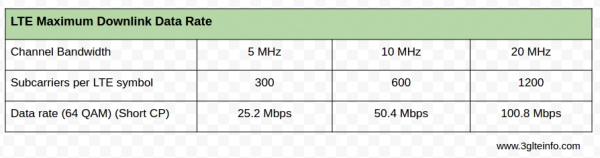# How to Calculate LTE Data Rate – Downlink Throughput

This tutorial explains how LTE downlink maximum throughput is determined. This is a simple and straightforward formula for data rate calculation.

The maximum data rate depends on channel bandwidth. As LTE uses different channel bandwidths both for FDD and TDD.

Let’s take the example for LTE using FDD, where channel bandwidth can be 5 MHz, 10 MHz and 20 MHz. In LTE release 8 there is no carrier aggregation, so let’s just consider simple cases.

Here we are calculating maximum data rate at the physical layer. The data rate always depends on the channel bandwidth. For example, in case of 5 MHz channel bandwidth, 300 subcarriers are used.

For a perfect idle condition 64 QAM can be used. That means each symbol is now allowed to carry 6 bits.

So the total bits carried by 300 subcarriers for the duration of a symbol is 300 X 6 = 1800 bits.

Again 1 symbol is of 71.4 microseconds for LTE. So the data rate is 1800 / 71.4 = 25.2 Mbps.

So the formula for calculating maximum data rate at physical layer is:

(Number of subcarriers X 6) / 71.4 microseconds

For 10 MHz using the same formula the maximum data rate in downlink is 50.4 Mbps and for 20 MHz it is 100.8 Mbps.1.Why TM6 is called spatial multiplexing though there is only one layer and no data rate gains as such from TM6

2.Hi! I have never seen such values for the throughput in LTE FDD. On a bandwidth of 20MHz it’s either 75Mbps in SISO or 150Mbps for MIMO 2×2. I don’t understand why the values you posted here are so different.

3.The calculation assumes all resource elements are available for PDSCH. But on average, approximately 25% are used for overhead channels PBCH, PDCCH, etc, so 75% of that number is in line what what you observed. You can also look at TS 36.213. The maximum transport block size in table 7.1.7.2.1-1 is 75376 bits per 1 ms TTI, which is 75 Mbps for SISO also.

4.These calculations are nonsense. In order to calculate the data rate one must take into consideration not only the symbol but the code rate. The maximum code rate is 1. This implies 1 data bit per symbol. The code rates are as shown below:

4ary 1/3
16ary 2/3
64ary 1

Hence, for 600 RE LTE carrier (10 MHz) the maximum data rate is one for each symbol which implies a maximum 64ary data rate of (600)14000=8.4Mbps.

5.good fundamental to study the datarate calculation.

6.Can you explain how throughput in LTE is enhanced?

7.Can you explain how to calculate PDSCH Throughput in LTE? If RB, MCS, and TB are available. Please explain step by step.

8.What would be the impact of carrier aggregation on throughput ?
Say for ex. if we have 20MHz band and we aggregate one more 20MHz band. Assuming the modulation scheme to be 64QAM , should the throughput be now 100.8*2 Mbps?

9.Carrier aggregation have own overheads (1-2%)

10.hi

if work with LTE band 3 BW =10MHZ , MIMO , max expected throughput per cell and per user …

11.How can we calculate UE throughput for 4×2 MIMO

12.Subcarrier in your example is considered as antenna?

13.Tmobile is starting a wifi plan via fiber feeded LTE antennas. What do you think the actual throughput will be for the farmer end user?